GFG App
Open AppBrowser
Continue

# Class 8 NCERT Solutions – Chapter 4 Practical Geometry – Exercise 4.4

### Question 1: Construct the following quadrilaterals.

DE = 4 cm
EA = 5 cm
AR = 4.5 cm
∠E = 60°
∠A = 90°

Solution:

Steps of construction:

Step 1: Draw a line segment DE of 4 cm.
Step 2: Construct an angle of 60° at E.
Step 3: From E draw an arc of 5 cm and intersect the arc with the angle line, mark the intersection point as A.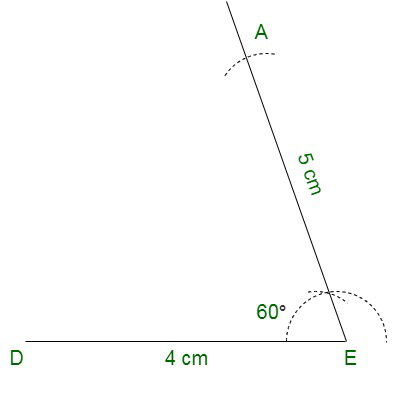Step 4: Draw a 90° angle at A.
Step 5: Draw an arc of 4.5 cm from A and cut the angle line at R.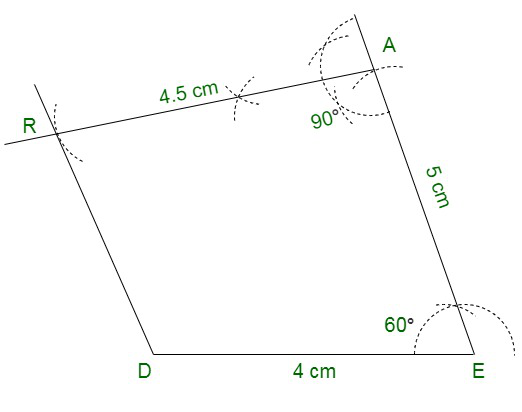Step 6: Join DR.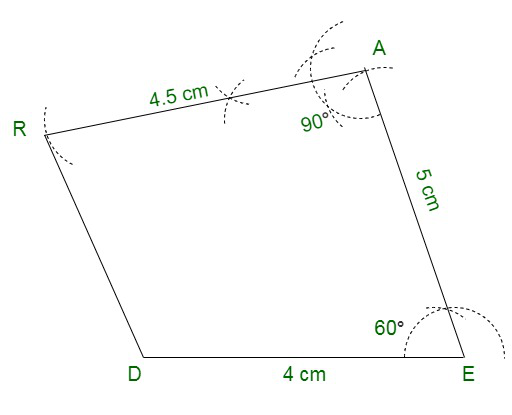Hence, we have the required quadrilateral DEAR.

TR = 3.5 cm
RU = 3 cm
UE = 4 cm
∠R = 75°
∠U = 120°

Solution:

Steps of construction:

Step 1: Draw a line TR of 3.5 cm.
Step 2: From R construct an angle of 75°.
Step 3: Draw an arc of 3 cm from R and cut the angle line at U.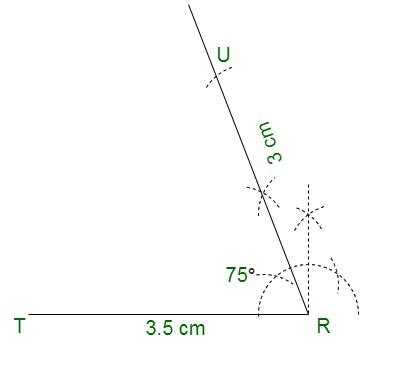Step 4: From U construct an angle of 120
Step 5: Draw an arc of 4 cm from U and cut the angle line at E.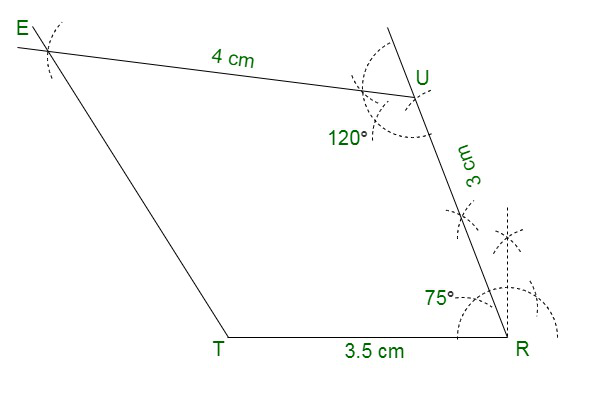Step 6: Join TE.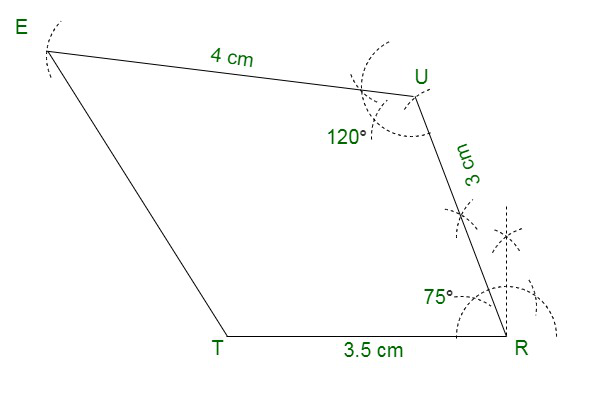Hence we have the required Quadrilateral TRUE.

My Personal Notes arrow_drop_up